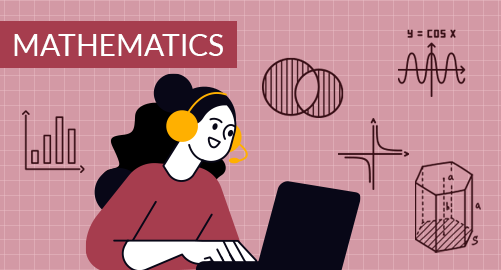#### Need Help?

Get in touch with usMathematics

Grade 4 Mathematics in the USA curriculum provides students with a solid foundation in critical mathematical skills and concepts, preparing them for more advanced mathematical adventures in the future. This grade level encompasses a variety of vital topics, including Number and Operations,Factors and Multiples , Fractions and Decimals, Geometry, Measurement, Data Analysis and Probability, Patterns and Algebraic Thinking, and emphasizes problem-solving skills for practical, real-world applications. As students advance through this curriculum, they will develop a deeper understanding of mathematical principles and problem-solving techniques, equipping them to apply math effectively in their daily lives

## What you'll learn

• 1

Number and Operations - Whole numbers , Integers, Add, Subtract , Multiply and Divide multidigit whole numbers,Estimating numbers

• 2

Factors and Multiples - Prime & Composite Numbers, Factors , Multiples .

• 3

Fractions and Decimals - Introducing to decimals, Place Value of decimals, Add , Subtract, Multiply and Divide fractions , Types of fractions , Simplifying fractions , Comparing and ordering decimals and fractions, Conversions .

• 4

Patterns and Algebra- Introduction to algebra , Number Sequences , Patterns

• 5

Geometry- Lines , Angles , Types of Angles, Patterns , Types of Symmetry , 2-D Shapes , 3-D Shapes, Nets of 3-D figures, Composite figures.

• 6

Measurements - Perimeter and Area of 2 D Shapes , Circumference and Area of Circles, Customary and Metric units of Length,

• 7

Data Handling and Probability - Organizing data, Pictographs, Bar graphs, Line graphs, Frequency charts , Measures of Central Tendency , Predicting Outcomes , Describing Probability

## Skills you'll learnNumeracy Skills - Distinguish between different types of numbers, Proficiency in fundamental arithmetic operations,Acquire the ability to estimate numbers.Problem Solving Skills - Understanding number relationships, Recognising prime and composite numbers, Simplifying complex problems,Recognizing number patterns and relationships.Arithematic Operations - Basic arithematic operations on Fractions, Decimals, Real world Problem Solving , Rounding Off and Computational Fluency.Algebraic Thinking - Understanding algebraic epxressions and equations , Recognise and apply the sequences, Identify PatternsGeometrical Skills and Spatial relationships - Proficiency in measuring angles , Properties of 2-D , 3-D Shapes , Create geometric patterns , Recognize symmetrical figures in the world around them , Visualize the relationships between 2-D shapes and 3-D objects.Measurement skills - Calculating the distance , Find the Space Occupied , Convert of units , Find the fence required through perimeter , Calculate the material required through areaData Analysis and Predictions - Data Organisation , Create graphs , Interpret data, Calculate Predictions, Calculate and measure of central tendency